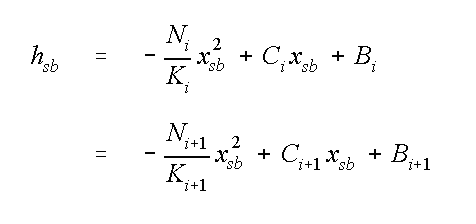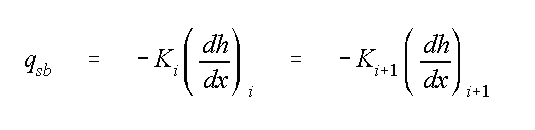Ecosystems Research

# EPA On-line Tools for Site Assessment Calculation

 17 of 67

#### Aquifers Composed of Multiple Segments

If an aquifer can be conceptualized as being composed of a set of segments, then different properties can be applied to each while still using the analytic solution given for the water table Bear (1972) In concept, this is the same idea that underlies the analytic element method Haitjema (1995) . Of particular interest are situations where the recharge varies over the site. By supplying differing recharge rates to segments of the model, the effects of locallized recharge variation can be incorporated into an analytic solution. The coefficients, C and B, which are determined for each segment, are chosen to satisfy continuity of head and flux as the segment boundaries are crossed. Since there can be no jump in head across segment boundaries,where xsb and hsb are the position and the head of the segment boundary, respectively. The flux condition is given bywhere qsb is the flux across the segment boundary. A consequence of this formulation is that there is a jump in gradient across the segment boundaries if the hydraulic conductivities differ. For each pair of segments there are two conditions that are applied where the segments join, and two conditions applied at exterior edges. Since the equations are linear, they can be solved using gaussian elimination.

 Previous Top ^ Next

Home | Glossary | Notation | Links | References | Calculators